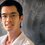Amazing problem!

Hello friends, We have to find the last two digits of $1\times3\times5\times7\times9....\times99$, I did and came out with 25.But I want to verify.Note by Kishan K
6 years, 3 months ago

This discussion board is a place to discuss our Daily Challenges and the math and science related to those challenges. Explanations are more than just a solution — they should explain the steps and thinking strategies that you used to obtain the solution. Comments should further the discussion of math and science.

When posting on Brilliant:

• Use the emojis to react to an explanation, whether you're congratulating a job well done , or just really confused .
• Ask specific questions about the challenge or the steps in somebody's explanation. Well-posed questions can add a lot to the discussion, but posting "I don't understand!" doesn't help anyone.
• Try to contribute something new to the discussion, whether it is an extension, generalization or other idea related to the challenge.
• Stay on topic — we're all here to learn more about math and science, not to hear about your favorite get-rich-quick scheme or current world events.

MarkdownAppears as
*italics* or _italics_ italics
**bold** or __bold__ bold

- bulleted
- list

• bulleted
• list

1. numbered
2. list

1. numbered
2. list
Note: you must add a full line of space before and after lists for them to show up correctly
paragraph 1

paragraph 2

paragraph 1

paragraph 2

> This is a quote
This is a quote
# I indented these lines
# 4 spaces, and now they show
# up as a code block.

print "hello world"
# I indented these lines
# 4 spaces, and now they show
# up as a code block.

print "hello world"
MathAppears as
Remember to wrap math in $$...$$ or $...$ to ensure proper formatting.
2 \times 3 $2 \times 3$
2^{34} $2^{34}$
a_{i-1} $a_{i-1}$
\frac{2}{3} $\frac{2}{3}$
\sqrt{2} $\sqrt{2}$
\sum_{i=1}^3 $\sum_{i=1}^3$
\sin \theta $\sin \theta$
\boxed{123} $\boxed{123}$

Sort by:

From the fact that it is divisible by $25$ but not by $2$, we can deduce that it ends with either $25$ or $75$. Now, consider the multiplications modulo $4$: $1 \times 3 \times 5 \times 7 \times 9 \times \dots \times 99 \equiv 1 \times 3 \times 1 \times 3 \times \dots \times 3 \equiv 1^{25} \times 3^{25} \equiv 1^{25} \times (-1)^{25} \equiv -1 \equiv 75 \not\equiv 25$, so the answer is $\boxed{75}$.

- 6 years, 3 months ago

Argh, you beat me to it xD Well done.

- 6 years, 3 months ago

how these type of shortcuts can be found? I want to know .if in any website it is plz tell me.

- 6 years, 2 months ago

I did not use any website. In fact, I never solved such a problem before. I guess you'll just need to be familiar with prime factors and the modulo operator.

- 6 years, 2 months ago

refer elemantary number theory by David M Burton

- 6 years, 2 months ago

I think you used Chinese remainder theorem.If not then please tell me the complete theorem.

- 6 years, 3 months ago

He did not use Chinese Remainder Theorem. Which part of his solution are you confused with?

- 6 years, 3 months ago

I am confused over the multiplication modulo 4...

- 6 years, 3 months ago

That is simply the individual remainders left when $4$ divides the given odd numbers which will obviously be $3$ or $1$ and you can easily find out the number of such terms which yield $1$ or $3$ as remainder.

- 6 years, 3 months ago

why only with 4, why not other?

- 6 years, 2 months ago

Because $4$ divides $100$, and looking at the last two digits of a number is essentially considering de remainder when that number is divided by $100$. As $4$ divides $100$, and we know that the number is one less than a multiple of $4$, then the last two digits of the number are also one less than a multiple of $4$. Hence, it's a nice trick to use here, as $75$ is one less than a multiple of $4$, and $25$ is not.

- 6 years, 2 months ago

thank you

- 6 years, 2 months ago

Its 75... courtesy mathematica ... :-P...Bt how do we solve these sort of problems is still a mystery to me..

- 6 years, 3 months ago

There is no theorem that says "oh, if you multiply the first 50 odd digits then the last 2 digits are 75." Rather, it takes intuition along with a knowledge of mathematical theorems. In this case, somebody figured "okay, well, there are more than 1 factors of "5" in this, but no factors of 2 so it must end in either 25 or 75. Then, to figure out if it was a 75 or 25 at the end, he counted the numbers of factors of 3, because any number ending in 25 when multiplied by 3 ends with 75, and any number ending in 75 multiplied by 3 ends with 25.

- 6 years, 3 months ago